# The box

The box contains five chocolate, three fruit, and two menthol candies. We choose sweets at random from the box. What is the probability that we will take out one chocolate, one fruit, and one menthol candy without a return?

Correct result:

p =  4.1667 %

#### Solution: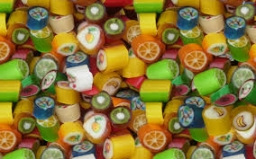We would be pleased if you find an error in the word problem, spelling mistakes, or inaccuracies and send it to us. Thank you!Tips to related online calculators
Would you like to compute count of combinations?

## Next similar math problems:

• Chambers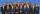The decision-making committee consists of three people. In order for the commission's decision to be valid, at least two members must vote in the same way. It is not possible not to vote in the commission, everyone only votes yes or no. We assume that the
• The universityAt a certain university, 25% of students are in the business faculty. Of the students in the business faculty, 66% are males. However, only 52% of all students at the university are male. a. What is the probability that a student selected at random in the
• The testThe test contains four questions, and there are five different answers to each of them, of which only one is correct, the others are incorrect. What is the probability that a student who does not know the answer to any question will guess the right answer
• Dices throwsWhat is the probability that the two throws of the dice: a) Six falls even once b) Six will fall at least once
• Hazard game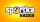In the Sportka hazard game, 6 numbers out of 49 are drawn. What is the probability that we will win: a) second prize (we guess 5 numbers correctly) b) the third prize (we guess 4 numbers correctly)?
• CardsFrom a set of 32 cards we randomly pull out three cards. What is the probability that it will be seven king and ace?
• Probability of intersectionThree students have a probability of 0.7,0.5 and 0.4 to graduated from university respectively. What is the probability that at least one of them will be graduated?
• Fall sum or sameFind the probability that if you roll two dice, it will fall the sum of 10, or the same number will fall on both dice.
• Two acesFrom a 32 card box we randomly pick 1 card and then 2 more cards. What is the probability that last two drawn cards are aces?
• Components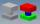In the box are 8 white, 4 blue and 2 red components. What is the probability that we pull one white, one blue and one red component without returning?
• Boys and girlsThere are 11 boys and 18 girls in the classroom. Three pupils will answer. What is the probability that two boys will be among them?
• Peaches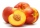There are 20 peaches in the pocket. 3 peaches are rotten. What is the probability that one of the randomly picked two peaches will be just one rotten?
• Alarm systems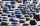What is the probability that at least one alarm system will signal the theft of a motor vehicle when the efficiency of the first system is 90% and of the independent second system 80%?
• Math logicThere are 20 children in the group, each two children have a different name. Alena and John are among them. How many ways can we choose 8 children to be among the selected A) was John B) was John and Alena C) at least one was Alena, John D) maximum one wa
• Phone numbersHow many 7-digit telephone numbers can be compiled from the digits 0,1,2,..,8,9 that no digit is repeated?
• BallsFrom the urn in which are 7 white balls and 17 red, gradually drag 3-times without replacement. What is the probability that pulls balls are in order: red red red?
• Pairs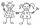From the five girls and four boys teachers have to choose one pair of boy and girl. A) How many such pairs of (M + F)? B) How many pairs where only boys (M + M)? C) How many are all possible pairs?